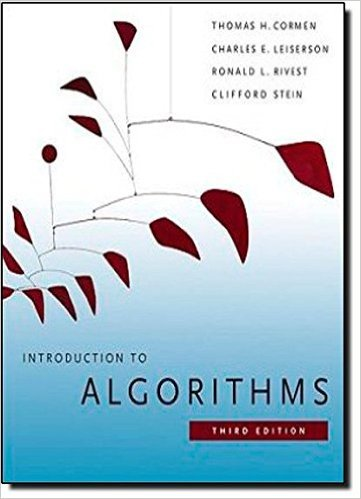×
Get Full Access to Introduction To Algorithms - 3 Edition - Chapter 24 - Problem 24-3
Get Full Access to Introduction To Algorithms - 3 Edition - Chapter 24 - Problem 24-3

×

# Arbitrage Arbitrage is the use of discrepancies inISBN: 9780262033848 130

## Solution for problem 24-3 Chapter 24

Introduction to Algorithms | 3rd Edition

• Textbook Solutions
• 2901 Step-by-step solutions solved by professors and subject experts
• Get 24/7 help from StudySoup virtual teaching assistantsIntroduction to Algorithms | 3rd Edition

4 5 1 343 Reviews
20
1
Problem 24-3

Arbitrage Arbitrage is the use of discrepancies in currency exchange rates to transform one unit of a currency into more than one unit of the same currency. For example, suppose that 1 U.S. dollar buys 49 Indian rupees, 1 Indian rupee buys 2 Japanese yen, and 1 Japanese yen buys 0:0107 U.S. dollars. Then, by converting currencies, a trader can start with 1 U.S. dollar and buy 49 2 0:0107 D 1:0486 U.S. dollars, thus turning a profit of 4:86 percent. Suppose that we are given n currencies c1; c2;:::;cn and an n n table R of exchange rates, such that one unit of currency ci buys Ri; j units of currency cj . a. Give an efficient algorithm to determine whether or not there exists a sequence of currencies hci1; ci2 ;:::;cik i such that Ri1; i2 Ri2; i3 Rik1; ik Rik; i1>1: Analyze the running time of your algorithm. b. Give an efficient algorithm to print out such a sequence if one exists. Analyze the running time of your algorithm. 24-4 Gabo

Step-by-Step Solution:
Step 1 of 3

Chapter 8: Matrices 8.2: Operations with matrices A matrix A is equalijo [a ] where i and j have to be numbers greater than 1. In order for a matrix to be equal to another its numbers a and its order (ij) have to be the same. For example: 1 7 = 7 9 5 In order for this two matrices to be equal x must be equal to 5 y equal to 1 and z equal to 9. Addition and subtraction Two matrices have to be the same in order for them to be added or subtracted from each other. To add or subtract a matrix you have to add or subtract the numbers from each order by the the same order of the other matrix. For example: 2 3 −3 7 A = , B =

Step 2 of 3

Step 3 of 3